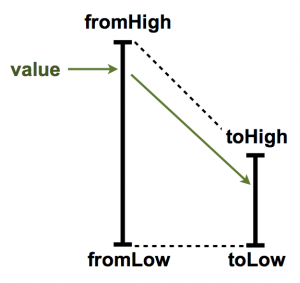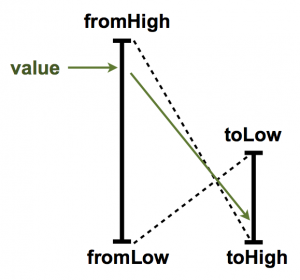# Sample Quiz Questions

You can email your answers to me (Kevin) anytime before Sunday, November 2nd at 11:59 if you would like verification that your answers are correct.

# DIY Website

http://www.kobakant.at/DIY/

# The Map Function

Here is a function that may be useful for many of the projects.

map(value, fromLow, fromHigh, toLow, toHigh)
http://arduino.cc/en/reference/map

Here is a diagram of how this function worksThe function takes a value that is in the range of fromHigh to fromLow.  It then remaps the value to toHigh to toLow.

One very nice applicatin of this technique is for remapping analog input to analog output.

void loop()
{
val = map(val, 0, 1023, 0, 255);
analogWrite(9, val);
}
Another interesting aspect of this function is that it allows us to flip the lows and highs as shown in the diagram below.This may be really useful for items such as the RGB LED that work by driving a signal low.

# Arduino Language Reference

Here is a link for the functions of the arduino programming language.

http://arduino.cc/en/Reference/HomePage

### Structure

#### Arithmetic Operators

• = (assignment operator)
•  (subtraction)
• * (multiplication)
• / (division)
• % (modulo)

#### Comparison Operators

• == (equal to)
• != (not equal to)
• < (less than)
• > (greater than)
• <= (less than or equal to)
• >= (greater than or equal to)

• && (and)
• || (or)
• ! (not)

#### Bitwise Operators

• & (bitwise and)
• | (bitwise or)
• ^ (bitwise xor)
• ~ (bitwise not)
• << (bitshift left)
• >> (bitshift right)

#### Compound Operators

• ++ (increment)
•  (decrement)
• -= (compound subtraction)
• *= (compound multiplication)
• /= (compound division)
• &= (compound bitwise and)
• |= (compound bitwise or)

# For off-campus website access (VPN)

To log into the website you need to have a campus IP.  If you are off-campus you can use VPN to obtain a campus IP.  Here are instructions on how to do so:http://www.doit.wisc.edu/network/vpn/